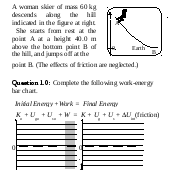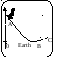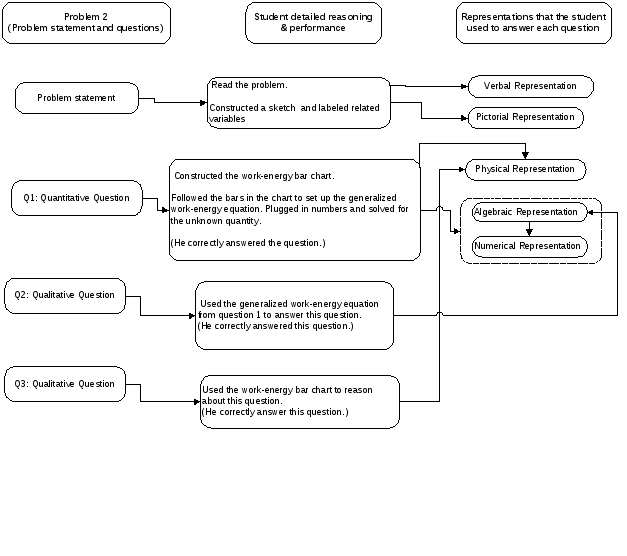# The role of work-energy bar charts as a physical representation in problem solving Xueli Zou

 The role of work-energy bar charts as a physical representation in problem solving Xueli Zou Department of Physics, California State University-Chico, Chico, California 95929-0202 An energy process can be represented by verbal, pictorial, bar chart, and mathematical representations. This multiple-representation technique has been introduced and used in the work-energy part of introductory college physics courses. Assessment indicates that the work-energy bar charts, as a physical representation of a work-energy process, play an important role in student problem solving: they help students 1) reason about work-energy problems conceptually first, 2) set up the generalized work-energy equation correctly and easily, and 3) make inferences and evaluate their problem solutions. One important goal of this investigation is to provide a research base for the design of instruction to help students develop expertise in solving work-energy problems. In the past thirty years, substantial progress in cognitive science, psychology, and physics education has been made in exploring and understanding differences in problem solving between experts and novices.1-10 The central difference in physics problem solving between experts and novices, according to Jill Larkin,4 is that novices have much less ability than experts to construct or use physical representations (e.g., free-body force diagrams). Few studies, however, investigate in depth what functions physical representations play in problem solving. This paper reports on a study of the role of work-energy bar charts7 as a physical representation in solving work-energy problems by introductory physics students. overview of the research The study was conducted through the analysis of responses to written questions from a large number of students and the analysis of think-aloud individual interviews11 with a small number of students. Subjects involved regular students, honors engineering freshmen, and honors physics majors from introductory calculus-based mechanics classes at The Ohio State University (OSU). Research results reported in this paper were primarily drawn from student interviews; on a voluntary basis, twelve honors engineering freshmen participated in pre-interviews and six honors engineering freshmen (not selected in the pre-interview) and four honors physics majors participated in follow-up interviews. By the time of the pre-interview, the students had been exposed to the concepts of kinematics and dynamics but not the concepts of work and energy (but all twelve students were exposed to the context of work and energy in their high school physics classes). Three problems (see Fig. 1) were used for the pre-interview, each basically including three small questions: Question 1 could be answered applying the conservation of energy quantitatively and Questions 2 and 3 were conceptual reasoning ones. In addition, the first interview problem (see Fig. 1) included a question asking the student to complete work-energy bar charts for the given situation, but in the two other problems completed work-energy bar charts were given. (A short tutorial on work-energy bar charts was given to the student at the beginning of the pre-interview.) Also, in all the three problems a picture was given to illustrate each physical situation. The pre-interview was used to investigate 1) how students solved work-energy problems from a perspective of problem representations, and 2) how they were be able to use a physical representation without formally learning how to use it in problem solving. In the follow-up interview three word problems were given (see Fig. 2), each including three small questions: Question 1 was a quantitative one, but Questions 2 and 3 were qualitative ones. No other problem representations were given in the problems. The follow-up interview was designed to study 1) how students applied work-energy bar charts in their problem solving, and 2) what functions the work-energy bar charts played as a physical representation. II. Pre-Interview ReSults Student solutions and interview videotapes from nine students were analyzed and transcribed. (Three other students did not finish all the three problems within theQuestion 1: What is the woman skier’s speed at B? Question 2: A male skier of mass 100 kg descends along the same hill. He starts from rest at point A as well, and jumps off at the bottom point B. Would his speed at point B be greater than, equal to, or less than the woman skier’s speed at point B? Explain your reasoning.Question 3: If the woman skier jumped off at point C that is above point B (see the figure at right), would her speed at point C be greater than, equal to, or less than her speed at point B? Explain your reasoning. Fig. 1: One of three problems used in individual student pre-interviews. one-hour pre-interview.) First, we found that for the first problem (see Fig. 1) eight of them correctly constructed work-energy bar charts that were consistent with the initial and final states that they chose. Second, only one student used the given work-energy bar charts to help himself answer the last interview problem, which was more difficult than the first two problems. But all the students used the given pictures to understand or infer the problems. Third, it was observed that all of the nine students, to some extent, just used numbers to answer the quantitative question in each problem, rather than setting up the generalized work-energy equations in a symbolic representation. In summary, we found that it was not difficult for students to understand the format and the structure of work-energy bar charts, but it was not natural for them to use the bar charts in their problem solving, compared to a sketch or picture. Beginning students used a numerical-representation- A spring of force constant 100 N/m is horizontally fixed to a smooth table. The top of the table is 1.0 m above the floor. The spring is initially compressed 0.2 m. After the spring is released, a HotWheelTM toy car of mass 0.05 kg is launched by the spring. After the toy car moves 0.5 m away from the spring, the car goes up a smooth ramp fixed to the same table top. The ramp horizontally makes an angle of 300 with the table top, and the ramp top is 0.8 m above the table top. After the toy car moves up the ramp, it flies off the ramp top, and eventually lands on the floor. (The effects of friction are neglected. g = 10 m/s2) Question 1: What is the speed of the toy car just before it hits the floor? Question 2: If the angle that the ramp makes with the table top is decreased, will the speed of the toy car, just before it lands on the floor, be the same, greater, or less than the speed in Question 1? Explain your reasoning. Question 3: If the height of the table is lowered, will the speed of the toy car, just before it lands on the floor, be the same, greater, or less than the speed in Question 1? Explain your reasoning. Fig. 2: One of the three problems used in individual student follow-up interviews. related concepts in numbers first, assemble these numbers together based on a law or a principle, and calculate a number for the Fig. 2: One of three problems used in individual student follow-up interviews. centered strategy in problem solving: represent related concepts in numbers first, assemble these numbers together based on a law or a principle, and calculate a number for the unknown variable. Using this naïve approach, students met difficulty conceptually understanding a physical process—the meaning of each concept became invisible or lost. III. Follow-up Interview Results After the honors engineering freshmen were exposed to two weeks of instruction on the concepts of work and energy using a multiple representation strategy—representing a work-energy process in verbal, pictorial, physical (work-energy bar charts) and mathematical representations (see Ref. 7 for details), six students from this class were interviewed. For comparison, we also interviewed four honors physics majors who had learned the concepts of work and energy in other approaches. The analysis of a solution for the problem shown in Fig. 2 by an honors engineering freshman was illustrated in Fig. 3. Based on students’ performance on the interview problems, we found that students who learned the multiple-representation strategy used this technique to solve the work-energy problems. In particular, the students used work-energy bar charts to help them understand the problems conceptually first, to set up the generalized work-energy equations, and to reason about some questions qualitatively. Problem solutions produced by the four honors physics majors, who learned the concepts of work and energy using other approaches, reflected some problem-solving expertise, such as working forward and recognizing the problems using the conceptsFig. 3. The analysis of a solution for the follow-up interview problem shown in Fig. 2 by an honors engineering freshman who learned the multiple-representation strategy in solving work-energy problems. of work and energy.1 But these students primarily used the numerical- representation-centered approach. Also, it was observed that the students went back and forth between pictorial representations (e.g., sketches or pictures) and numerical representations to reason about the problems. Although a picture or a sketch could help students visualize and infer a physical process,8 it included real objects such as springs, inclined planes, and others—often triggering students’ responses based on surface features. It appeared that without being able to access a physical representation, it is difficult for students to understand and infer a problem conceptually before they used equations. IV. Conclusion In summary, the study can show that work-energy bar charts play an important role in student problem solving: 1) They help students use the concepts of work and energy first to qualitatively reason about the problem, rather than identifying the problem based on surface features. 2) They help students set up the generalized work-energy equations correctly and easily. A completed work-energy bar chart provides visual aid for students to construct the mathematical equation—there is one term in the equation for each bar in the bar chart. 3) They direct students to make inferences and allow them to evaluate their problem solutions. Being independent of surface features of real objects and in a bar graph format, work-energy bar charts aid students in “seeing” the conservation of energy easily and in assessing their solutions effectively. Acknowledgments I gratefully thank Alan Van Heuvelen and the OSU Physics Education Research Group for their valuable help with this study. Larkin, J., McDermott, J., Simon, D. P., and Simon, H. A., “Expert and novice performance in solving physics problems,” Science 208, 1335-1362 (1980). F. Reif and J. I. Heller, "Knowledge structures and problem solving in physics," Ed. Psych. 17, 102-127 (1982). J. I. Heller and F. Reif, "Prescribing effective human problem-solving processes: Problem description in physics," Cog. Instr. 1, 177-216 (1984). Fig. 3. An example of solutions for Problem 2 (see Fig. 2) used in the follow-up interview by the honors physics major who did not learn the work-energy bar chart strategy. J. Larkin, "Understanding, problem representations, and skill in Physics," In S. F. Chipman, J. W. Segal & R. Glaser (eds), Thinking and Learning Skills (Lawrence Erlbaum Associates, Hillsale, NJ, 1985), Vol. 2, pp. 141-159. J. Larkin, "The role of problem representation in physics," In D. Gentner and A. L. Stevens (eds), Mental Models (Lawrence Erlbaum Associates, Hillsale, NJ, 1983), pp. 75-98. A. Van Heuvelen, "Learning to think like a physicist: A review of research-based instructional strategies," Am. J. Phys. 59, 891-897 (1991). A. Van Heuvelen and X. Zou "Multiple representations of work-energy processes" Am. J. Phys. 69, 184-194 (2001). J. Larkin and H. Simon, "Why a diagram is (sometimes) worth ten thousand words,” Cog. Sci. 11, 65-99 (1987). Chi, M. T. H., Feltovich, P. J., and Glaser, R., “Categorization and representation of physics knowledge by expert and novices,” Cog. Sci. 5, 121-152 (1981). Zhang, J., “The nature of external representations in problem solving,” Cog. Sci. 21, 179-217 (1997). Ericsson, K. A. and Simon, H. A., Protocol analysis: Verbal report as data (The MIT Press, Cambridge, Massachusetts, 1996). Share with your friends: